Get instant live expert help with Excel or Google Sheets“My Excelchat expert helped me in less than 20 minutes, saving me what would have been 5 hours of work!”

#### Post your problem and you’ll get expert help in seconds.

Your message must be at least 40 characters
Our professional experts are available now. Your privacy is guaranteed.

# How to prepare cash flow statement – Excelchat

We can prepare a Cash Flow Statement by using the cash flow template given in this article. This tutorial will guide all levels of excel users on how to create a simple cash flow template to calculate statement of cash flow.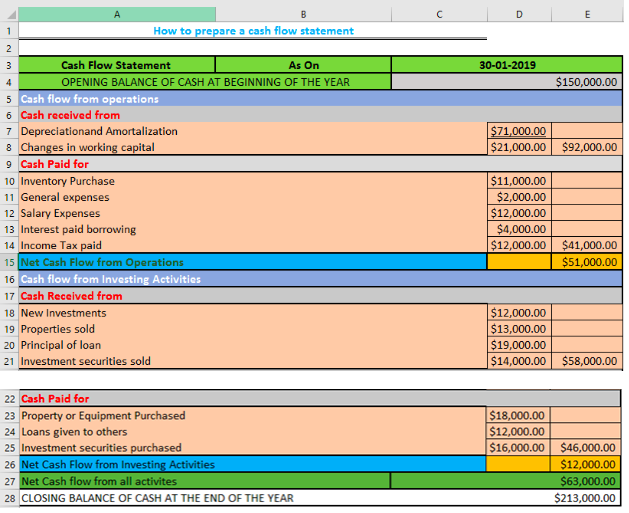Figure 1: Cash Flow Statement Template

## Setting the data

• To do this, we will input all the titles of our expenditure in their various activities. This tutorial has a cashflow from two activities, “cash flow from operations” and “cash flow from investing activities”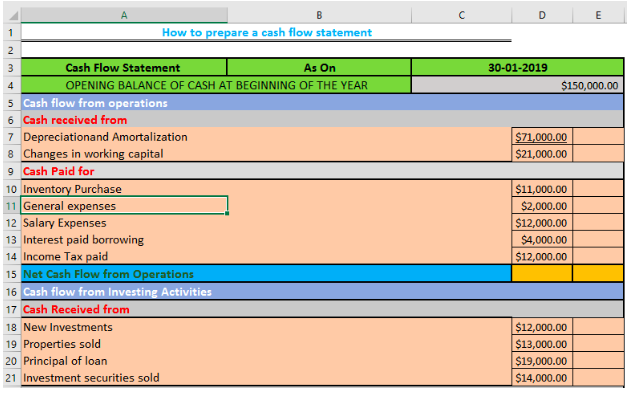Figure 2: Title of Expenditure

• We will now calculate the sum of the cash received from and cash paid for both the cash flow from Operations and cash flow from investing activities by typing into Cells E8, E14 and E21 the formula “`=SUM(D7:D8)`, `=SUM(D10:D14)`, and `=SUM(D18,D21)`, and then press the enter key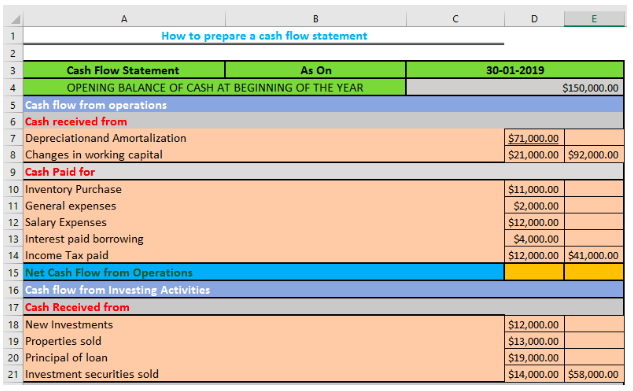Figure 3: Sum of Cash Flow Operations

• We will now calculate the net cash flow from operations by subtracting the cash paid for from cash paid from by typing the formula below into Cell E15

`=Sum(E8 -``E14)` or `=(E8 ``-E14)`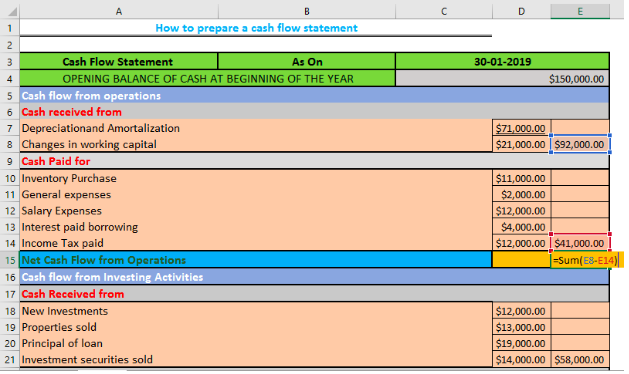Figure 4: Net cash flow from operations

• We will repeat also for Cell E26 for the net cash flow from investing activities

`=Sum(E21-E25)`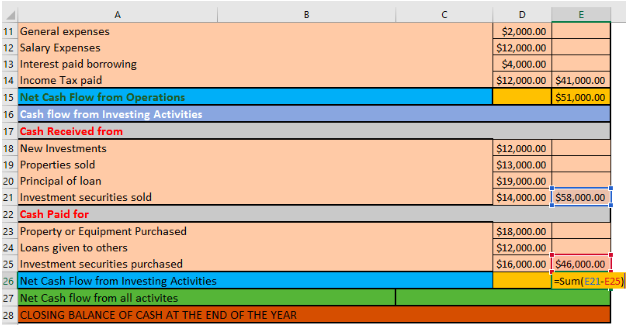Figure 5: Net cash flow from investing activities

• We will now calculate the net cash flow from all activities by adding up all the net cash flows using this formula, “`=SUM(E15+E26)`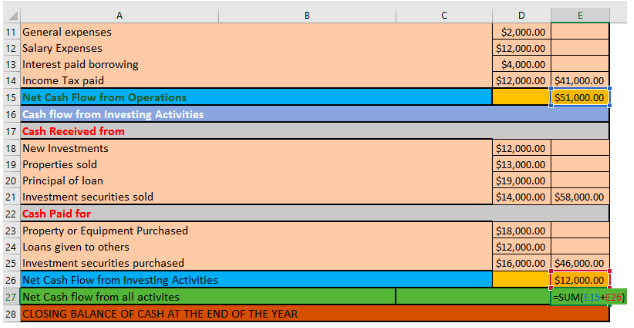Figure 6: Sum of all Net Cash Flows

• We will now close the balance by typing in this formula into Cell E28

`=SUM(E27+C4)`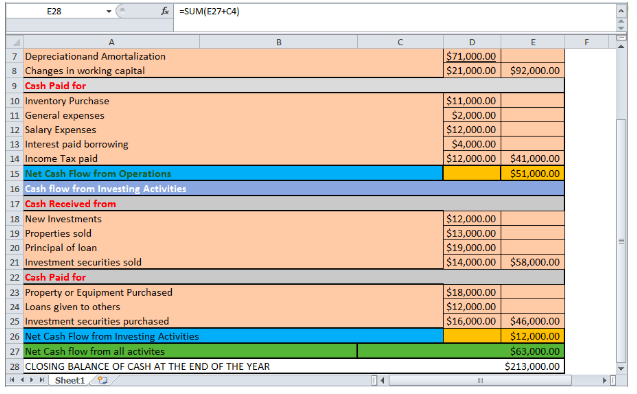Figure 7: Closing balance

## Note

• Cash Flow Statement Components
This provides a detailed view of cash from operations and Investing Activities

• Cash Flow from Operating Activities
The net amount coming in or going out on daily business operations

• Cash Flow from Investing Activities
The outflow of cash for long-term assets such as equipment, buildings and landed properties

## Instant Connection to an Expert through our Excelchat Service

Most of the time, the problem you will need to solve will be more complex than a simple application of a formula or function. If you want to save hours of research and frustration, try our live Excelchat service! Our Excel Experts are available 24/7 to answer any Excel question you may have. We guarantee a connection within 30 seconds and a customized solution within 20 minutes.